# p5.js临摹旋转爱心_茜茜_前端开发者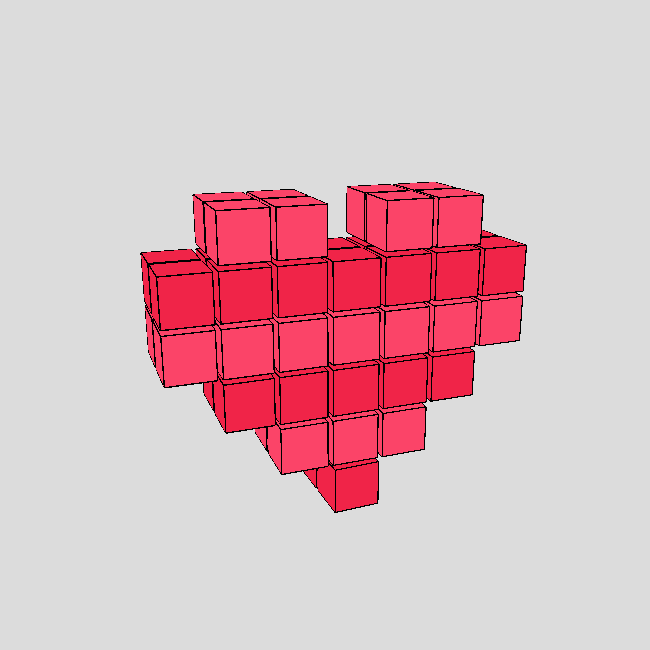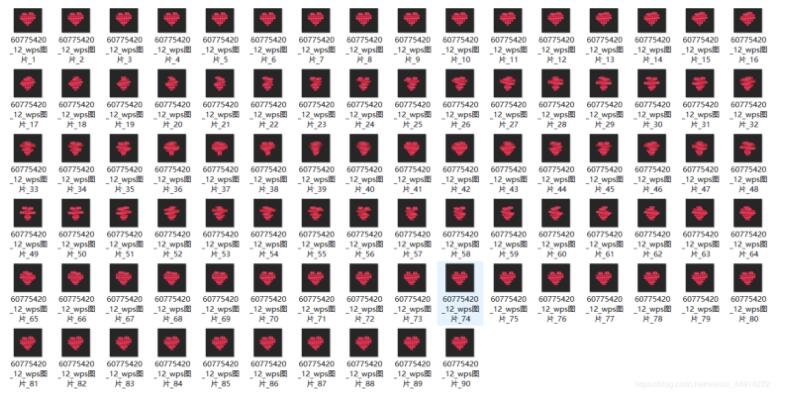（注：使用时记得转化为弧度制。）

```function setup() {
createCanvas(windowWidth, windowHeight,WEBGL);
}

function draw() {
background(220);
}```

```function drawHeart1(BoxSize,posX,posY,posZ,r,g,b)  //第一排方块
{
fill(r,g,b);
translate(posX-(10/9)*BoxSize,posY,posZ);
box(BoxSize);
translate(-(10/9)*BoxSize,0,0);
box(BoxSize);
translate(3*(10/9)*BoxSize,0,0);
box(BoxSize);
translate((10/9)*BoxSize,0,0);
box(BoxSize);
translate(0,0,(10/9)*BoxSize);
box(BoxSize);
translate(-(10/9)*BoxSize,0,0);
box(BoxSize);
translate(-2*(10/9)*BoxSize,0,0);
box(BoxSize);
translate(-(10/9)*BoxSize,0,0);
box(BoxSize);
}```

drawHearts（）中第一个参数是立方体的边长，第二到第四个参数是XYZ轴的偏移量，最后三个为立方体颜色RGB值。

```push();
translate(0,0);
drawHeart1(40*size1,0,-2*40*10/9,-20,251,68,104);
pop();```①设置旋转角度变量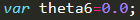②在draw()函数中关于第六层代码。其中theta6-=360*PI/180/90表示一帧旋转的弧度增量。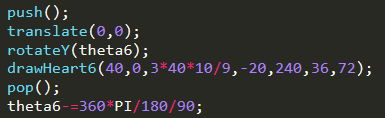②在draw()函数中关于第五层代码。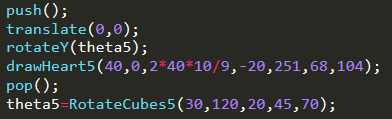③RotateCubes5()函数。第一个参数表示逆时针旋转角度，第二个参数表示顺时针旋转角度的二分之一，第四、五、六个参数用来判断旋转时帧的范围，并用于计算旋转角度。

```function RotateCubes5(delta1,delta2,f1,f2,f3){
if(frameCount%90>=1&&frameCount%90<=f1)
{
theta5-=(f1-frameCount%90)*delta1*PI/180/Summation(0,f1-1);
}
if(frameCount%90>=f1+1&&frameCount%90<=f2)
{
theta5+=(frameCount%90-f1-1)*delta2*PI/180/Summation(0,f2-f1-1);
}
if(frameCount%90>=f2+1&&frameCount%90<=f3)
{
theta5+=(f3-frameCount%90)*delta2*PI/180/Summation(0,f2-f1-1);
}
if(frameCount%90>=f3+1&&frameCount%90<=89)
{
theta5-=(frameCount%90-f3-1)*delta1*PI/180/Summation(0,f1-1);
}
if(frameCount%90==0)
{
theta5-=(90-f3-1)*delta1*PI/180/Summation(0,f1-1);
}
return theta5;
}```

③Summation()函数，即高斯求和。

```function Summation(n1,n2)
{
return (n1+n2)*(n2-n1+1)/2;
}```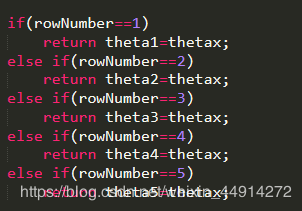```if(frameCount%200>=0&&frameCount%200<=99)  //扩展作品2
{
size1=frameCount%100*0.02;
size2=(100-frameCount%100)*0.02;
size3=frameCount%100*0.02;
size4=(100-frameCount%100)*0.02;
size5=frameCount%100*0.02;
size6=(100-frameCount%100)*0.02;
}
if(frameCount%200>=100&&frameCount%200<=199)
{
size1=(100-frameCount%100)*0.02;
size2=frameCount%100*0.02;
size3=(100-frameCount%100)*0.02;
size4=frameCount%100*0.02;
size5=(100-frameCount%100)*0.02;
size6=frameCount%100*0.02;
}```

» 本文来自：前端开发者 » 《p5.js临摹旋转爱心_茜茜_前端开发者》
» 本文链接地址：https://www.rokub.com/70947.html
» 您也可以订阅本站：https://www.rokub.com
64K

• 回顶